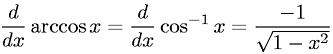Equations > Calculus > Differentiation/Differentals > Derivative of Inverse Cosine (Arccosine)

### Derivative of Inverse Cosine (Arccosine)Latex Code:

MathML Code:

 $\fracd\mathrm{dx}\mathrm{arccos}x=\fracd\mathrm{dx}{\mathrm{cos}}^{-1}x=\frac-1\sqrt{1-{x}^{2}}$

MathType 5.0: